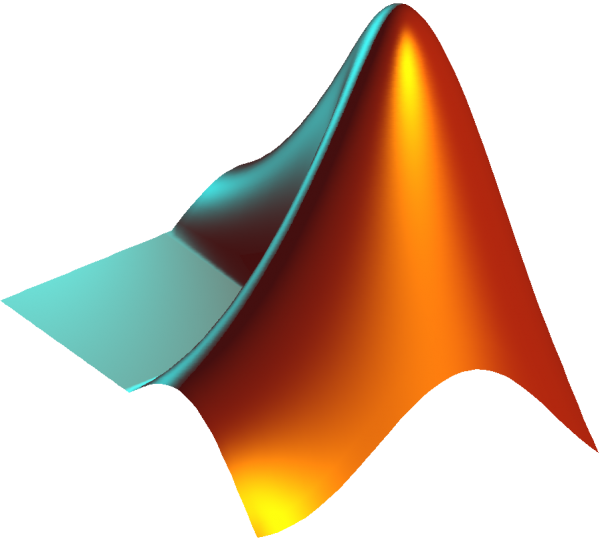News

# Importance of MATLAB to Math StudentsWritten by
You Are Here » Home » News» Importance of MATLAB to Math StudentsMath is arguably the most challenging subject that most students struggle to comprehend. However, with innovative products in today’s digitally-driven world, math continuously proves fun and manageable. Here is the best part; you can also learn at your pace, especially with professional help from Adeptassignmentdoers. As students endeavor to hone their math skills, they have an extensive pool of resources to help fast-track the process. From libraries and online forums to the readily available tools, you have a rich collection that can supercharge your math skills. Among the must-have tools for math students includes MATLAB.

Developed by MathWorks, MATLAB is a multi-paradigm programming language that offers a numeric computing environment. Among the top areas that make MATLAB an ideal math tool include illustrations of the order of operations, quadratic equations, factoring, operations with polynomials, linear equations, to mention a few. You can solve a range of problems in a portion of the time it would take to handle without MATLAB, and with outstanding features, learn math with ease. As MATLAB integrates programming, computation, and visualization in an easy-to-use environment, addressing problems and providing solutions in familiar math notation, it comes in handy in various ways, including;

# Statistics

Among the tedious and challenging math elements are statistics. From the data analysis, exploration, and visualization, statistical concepts can quickly take a toll on your progress. MATLAB offers an extensive library that makes the process easier. As you handle statistics with MATLAB, the principal functions that speed up the process while enhancing your work’s dependability include simulations, prototyping, and modeling.  With practical visualization functions, you can comfortably handle an extensive database without affecting your processes’ accuracy as most of the tedious and challenging tasks are eased.  Application development lets you take advantage of graphical user interface building to enhance visualization efforts. With scientific and engineering graphics, you can comfortably handle a range of statistical concerns without hitting a nag after another, stressing the need to use MATLAB in your math quests.

# Basic math computations

Math prowess considerably depends on your basic computation comprehension. The primary computations, such as knowing where to start while dealing with two or more operations in an expression, might seem to flow naturally, but this is as you’ve repeatedly followed PEMDAS, meaning that you no longer have to revisit it to establish where to begin and what comes next. With MATLAB, you can quickly understand basic math computations going beyond PEMDAS (parenthesis, exponents, multiplication, division, addition, subtraction). From the linear equation system to operations with polynomial, MATLAB makes learning more engaging as you use the illustrative functions. This makes it easier to master basic math computations, establish a strong foundation, and build on your math prowess to handle even the most challenging areas.

# Algorithms

Algorithms are an integral part while learning math. You can learn how to use MATLAB as an advanced calculator. It gets better; you can develop your algorithm. Developing, implementing, and testing algorithms are more accessible with MATLAB. You can comfortably create computational codes to handle various problems. Leveraging an extensive database of built-in algorithms, you can develop a solid system for all your math problems. Debugging is also easy, saving more time while delivering dependable solutions.

MATLAB is easy to use, and with a rich support system, including books, online forums, among others, you can hone your skis as you endeavor to improve your math performance. MATLAB teaches you how to solve various math problems with a code. As you strive to leverage tech advancements in your math learning quest, MATLAB skills come in handy in more ways than you might initially anticipate, making an excellent addition for students.

### About the author